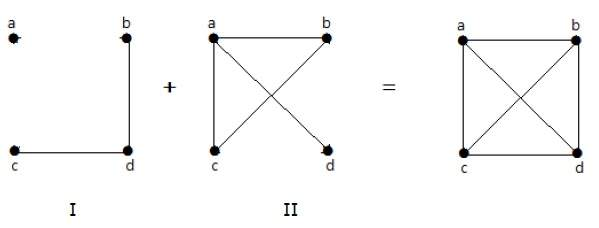# Complement of Graph

MathematicsComputer EngineeringMCA

Let 'G' be a simple graph with some vertices as that of 'G' and an edge {U, V} is present in 'G', if the edge is not present in G. It means, two vertices are adjacent in 'G' if the two vertices are not adjacent in G.

If the edges that exist in graph I are absent in another graph II, and if both graph I and graph II are combined together to form a complete graph, then graph I and graph II are called complements of each other.

## Example

In the following example, graph-I has two edges 'cd' and 'bd'. Its complement graph-II has four edges.Note that the edges in graph-I are not present in graph-II and vice versa. Hence, the combination of both the graphs gives a complete graph of 'n' vertices.

Note − A combination of two complementary graphs gives a complete graph.

If 'G' is any simple graph, then

|E(G)| + |E('G-')| = |E(Kn)|, where n = number of vertices in the graph.

## Example

Let 'G' be a simple graph with nine vertices and twelve edges, find the number of edges in 'G-'.

You have, |E(G)| + |E('G-')| = |E(Kn)|

12 + |E('G-')| =

9(9-1)/2 = 9C2

12 + |E('G-')| = 36

|E('G-')| = 24

'G' is a simple graph with 40 edges and its complement 'G' has 38 edges. Find the number of vertices in the graph G or 'G'.

Let the number of vertices in the graph be 'n'.

We have, |E(G)| + |E('G-')| = |E(Kn)|

40 + 38 =  n(n-1) / 2

156 = n(n-1)

13(12) = n(n-1)

n = 13